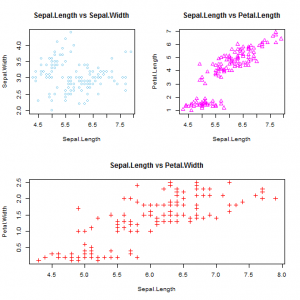Want to share your content on R-bloggers? click here if you have a blog, or here if you don't.In many situations, we require that several plots are placed in the same figure as subplots. R has various ways of doing it. Base Graphics has three different ways to draw subplots, i.e. ` mfrow`, `layout` and `split.screen`, with increasing degree of complexity, and, at the same time, with increased control over the plot elements. This example introduces the `mfrow``mfcol` and `layout` functions in Base Graphics. We use the familiar `iris` dataset for the illustrations.

Answers to the exercises are available here.If you obtained a different (correct) answer than those listed on the solutions page, please feel free to post your answer as a comment on that page.

Exercise 1
Consider the `iris` dataset, draw the following scatterplots, a) `Sepal.Length` vs `Sepal.Width`, b) `Sepal.Length ` vs `Petal.Length `, and c) `Sepal.Length ` vs `Petal.Width `. Annotate each scatterplot with a title. Use separate colors and plotting characters for each plot.

Exercise 2
Plot the three scatterplots in the same figure as subplots arranged in one row. Use `mfrow`.

Exercise 3
Plot the three scatterplots in the same figure as subplots arranged in one column. Use `mfrow` .

Exercise 4
Repeat the same scatterplots. Partition in such a way that the first row contains plots a and b, and the second row contain plot c. Use `mfrow`.

Exercise 5
Repeat Exercise 2 with `mfcol`.

Exercise 6
Repeat Exercise 3 with `mfcol`.

Exercise 7
Repeat Exercise 4 with `mfcol`.

Exercise 8
Repeat Exercise 2 with `layout`.

Exercise 9
Repeat Exercise 3 with `layout`.

Exercise 10
Repeat Exercise 4 with `layout`. In this case, let scatterplot c occupy the second row completely.